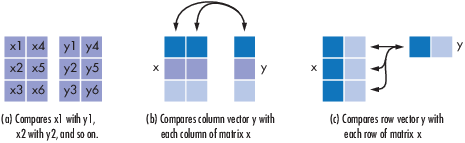# symerr

Compute number of symbol errors and symbol error rate

## Syntax

``[number,ratio] = symerr(x,y)``
``[number,ratio] = symerr(x,y,flg) ``
``[number,ratio,loc] = symerr(...)``

## Description

example

````[number,ratio] = symerr(x,y)` compares the elements in `x` and `y`. The sizes of `x` and `y` determine which elements are compared. The output `number` is a scalar or vector that indicates the number of elements that differ. The output `ratio` equals `number` divided by the total number of elements in the smaller input. ```
````[number,ratio] = symerr(x,y,flg) ` compares the elements in `x` and `y`. Optional input `flg` and the size of x, and y, determine the size of `number`.```

example

````[number,ratio,loc] = symerr(...)` returns a binary matrix `loc` that indicates which elements of `x` and `y` differ. An element of `loc` is zero if the corresponding comparison yields no discrepancy, and one otherwise.```

## Examples

collapse all

Compare Elements of Matrix with Another Matrix

`x = [1,1,3,1;3,2,2,2;3,3,8,3]`
```x = 3×4 1 1 3 1 3 2 2 2 3 3 8 3 ```
`aMatrix = [1,1,1,1;2,2,2,2;3,3,3,3]`
```aMatrix = 3×4 1 1 1 1 2 2 2 2 3 3 3 3 ```
`[number1,ratio1] = symerr(x,aMatrix)`
```number1 = 3 ```
```ratio1 = 0.2500 ```

Compare Elements of Matrix with Row Vector

`x = [1,1,3,1;3,2,2,2;3,3,8,3]`
```x = 3×4 1 1 3 1 3 2 2 2 3 3 8 3 ```
`aRowVector = [1,2,3,1]`
```aRowVector = 1×4 1 2 3 1 ```
`[number2,ratio2] = symerr(x,aRowVector)`
```number2 = 3×1 1 3 4 ```
```ratio2 = 3×1 0.2500 0.7500 1.0000 ```

Compare Elements of Matrix with Column Vector

`x = [1,1,3,1;3,2,2,2;3,3,8,3]`
```x = 3×4 1 1 3 1 3 2 2 2 3 3 8 3 ```
`aColumnVector = [1;2;3]`
```aColumnVector = 3×1 1 2 3 ```
`[number3,ratio3] = symerr(x,aColumnVector)`
```number3 = 1×4 1 0 2 0 ```
```ratio3 = 1×4 0.3333 0 0.6667 0 ```

You can specify alternative comparison methods used by `symerr`. In this example, you use a flag to override the default row-by-row comparison. Notice that number and ratio are scalars.

```format rat; [number,ratio,loc] = symerr([1 2; 3 4],[1 3],'overall')```
```number = 3 ```
```ratio = 3/4 ```
```loc = 0 1 1 1 ```

## Input Arguments

collapse all

First input to compare, specified as a vector, or a matrix.

Data Types: `double`

Second input to compare, specified as a vector, or a matrix.

Data Types: `double`

Optional argument to override the defaults that govern which elements `symerr` compares and how `symerr` computes the outputs.

• `'overall'` –– `x` and `y` are compared element by element.

• `'column-wise'` –– mth row of `x` vs. mth row of `y`.

• `'row-wise'` –– mth column of `x` vs. mth column of `y`.

## Output Arguments

collapse all

Number of elements that differ between `x` and `y`, returned as a scalar or vector. The size of `number` is determined by the optional input `flg` and by the dimensions of `x` and `y`. See Default Element Comparison and Specifying Element Comparison for more information.

The ratio of the number of differing elements, `number`, and the total number of elements of the smaller input, returned as a scalar.

Logical array of same size and dimensions as `x`, and `y`, with corresponding locations of errors between inputs `x` and `y`. An element of `loc` is zero if the corresponding comparison yields no discrepancy, and one otherwise.

collapse all

### Default Element Comparison

The `symerr` function compares binary representations of elements in `x` with those in `y`. When optional argument `flg` is not specified, `symerr` uses the shape of the inputs `x` and `y` to determine the element comparison method.

The schematics below illustrate how the shapes of `x` and `y` determine which elements `symerr` compares:• If `x` and `y` are matrices of the same dimensions, then `symerr` compares `x` and `y` element by element. `number` is a scalar. See schematic (a) in the figure.

• If one is a row (respectively, column) vector and the other is a two-dimensional matrix, then `symerr` compares the vector element by element with each row (resp., column) of the matrix. The length of the vector must equal the number of columns (resp., rows) in the matrix. `number` is a column (resp., row) vector whose mth entry indicates the number of elements that differ when comparing the vector with the mth row (resp., column) of the matrix. See schematics (b) and (c) in the figure.

### Specifying Element Comparison

Use `flg` to override the defaults that govern which elements `symerr` compares and how `symerr` computes the outputs. The values of `flg` are `'overall'`, `'column-wise'`, and `'row-wise'`. The table below describes the differences that result from various combinations of inputs. In all cases, `ratio` is `number` divided by the total number of elements in `y`.

Comparing a Two-Dimensional Matrix x with Another Input y

Shape of y`flg`Type of Comparisonnumber
Two-dim. matrix `'overall'` (default) Element by element Total number of symbol errors
`'column-wise'`mth column of `x` vs. mth column of `y` Row vector whose entries count symbol errors in each column
`'row-wise'`mth row of `x` vs. mth row of `y` Column vector whose entries count symbol errors in each row
Column vector `'overall'` `y` vs. each column of `x` Total number of symbol errors
`'column-wise'` (default) `y` vs. each column of `x` Row vector whose entries count symbol errors in each column of `x`

Row vector

`'overall'``y` vs. each row of `x` Total number of symbol errors
`'row-wise'` (default) `y` vs. each row of `x` Column vector whose entries count symbol errors in each row of `x`